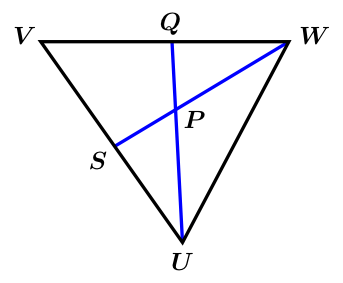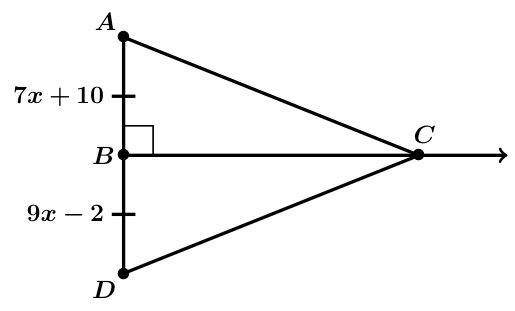# GEOM 2 | Lesson 4 | PracticeApply your knowledge of these terms in the following problems :

1. Solve for XY.$$\overline{WZ}=24$$

$$\overline{ZY}=12$$

$$\overline{WX}=30$$

$$\overline{XY}=$$ ?

1. In order for the red line to be the perpendicular bisector of $$\overline{AC}$$, what would the coordinates of the point D (where the red line intersects $$\overline{AC}$$, need to be? What would be the length of AD and DC?1. Find QV if $$QV=7x-1$$ and $$QW=4x+5$$, $$Q$$ is the midpoint of $$\overline{VW}$$A) 13         B) 19.5

C) 8.67       D) 26